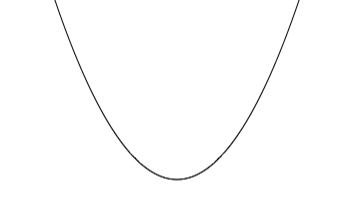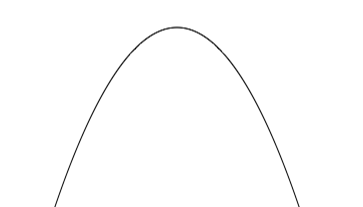SEARCH HOMEMath Central Quandaries & QueriesQuestion from Thomas, a parent: sketch a quadratic function with zeros at -3 and 1Hi Thomas,

The graph of a quadratic function is a parabola that either opens upwardsor downwards.If it has zeros at -3 and 1 then it crosses the X-axis at x = -3 and x = 1.

I hope this helps,
PennyMath Central is supported by the University of Regina and The Pacific Institute for the Mathematical Sciences.